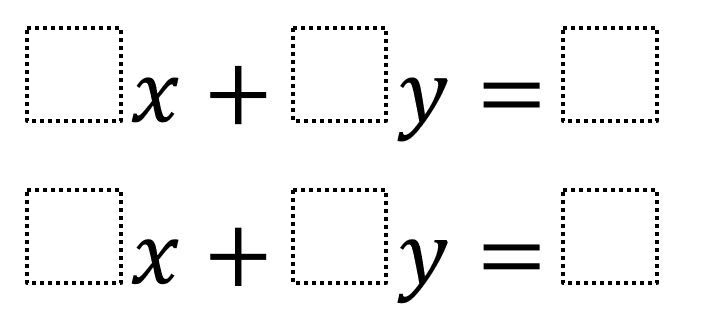Home > Grade 8 > Expressions & Equations > Equations of Perpendicular Lines

# Equations of Perpendicular Lines

Directions: Using the integers -9 to 9 (excluding 0) at most one time each, fill in the blanks to create two distinct perpendicular lines.### Hint

Why might we want to consider the relationship between the coefficients of x and y?

There are many solutions but not infinite

1 x + 2 y = 4
-6 x + 3 y = 5

2 x + 3 y = 9
-6 x + 4 y = 8

Any solution where the x and y coefficients swap and one is negative. Students will need to use multiples to avoid repeating values. The constant term can take any remaining value.

Source: Louise Pepper with answers from the students of Kings College Alicante, Spain

## Scientific Notation 2

Directions: Using the digits 1 to 9, at most one time each, fill in the …

1.2.Anyone else bored with online school?

3.Danitza Marenco

I got 2x+6y=9
-8+4y=8

4.1x +2y =4
3x+-6=5